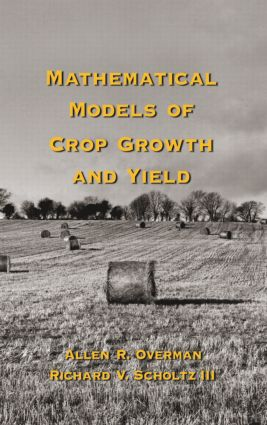# Mathematical Models of Crop Growth and Yield

## 1st Edition

CRC Press

344 pages

Paperback: 9780367395896
pub: 2019-09-05
\$74.95
x
Hardback: 9780824708252
pub: 2002-08-27
\$155.00
x

FREE Standard Shipping!

### Description

Highlighting effective, analytical functions that have been found useful for the comparison of alternative management techniques to maximize water and nutrient resources, this reference describes the application of viable mathematical models in data analysis to increase crop growth and yields. Featuring solutions to various differential equations, the book covers the characteristics of the functions related to the phenomenological growth model. Including more than 1300 literature citations, display equations, tables, and figures and outlining an approach to mathematical crop modeling, Mathematical Models of Crop Growth and Yield will prove an invaluable resource.

INTRODUCTION. Historic Background. Yield Response Models. Growth Models. Environmental Input. Summary. Exercises. References. SEASONAL RESPONSE MODELS. Background. Extended Logistic Model. Extended Multiple Logistic Model. Water Availability. Legume/Grass Interaction. Summary. Exercises. References. GROWTH RESPONSE MODELS. Background. Empirical Growth Model. Extended Empirical Growth Model. Phenomenological Growth Model. Expanded Growth Model. Exercises. References. MATHEMATICAL CHARACTERISTICS OF MODELS. Background. Phenomenological Growth Model. Expanded Growth Model. Rational Basis for Logistic Model. Coupling Among Applied Soil and Plant Components. Accumulation of Dry Matter and Plant Nutrients. Exercises. References. PASTURE SYSTEMS. Background. Quadratic Model. Linear Exponential Model. Summary. Exercises. References. NONLINEAR REGRESSION FOR MATHEMATICAL MODELS. Background. Logistic Model. Probability Model. Confidence Contours. Sensitivity Analysis. Dimensionless Plots. Correlation Coefficient. Exercises. References. Index.

Overman, Allen R.; Scholtz III, Richard V.